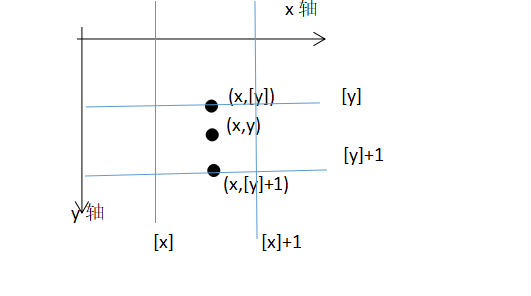# OpenCV实现图像变换（python）-仿射变换原理

2019/04/10 10:10

$\left( \begin{matrix} \overline{x} \ \overline{y} \end{matrix} \right) =\left( \begin{matrix} a_{11} &&a_{12} \ a_{21}&&a_{22} \end{matrix} \right) \left( \begin{matrix} x \ y \end{matrix} \right) + \left( \begin{matrix} a_{13} \ a_{23} \end{matrix} \right)$ 在以下矩阵中： $\left( \begin{matrix} \overline{x} \ \overline{y} \ 1 \end{matrix} \right) =A \left( \begin{matrix} x \ y \ 1 \end{matrix} \right)$ 矩阵A就是仿射矩阵，因为它最后一行为（0，0，1） $A= \left( \begin{matrix} a_{11}&a_{12}&a_{13} \ a_{21}&a_{22}&a_{23} \ 0&0&1 1 \end{matrix} \right)$ 平移 平移是最简单的仿射变换如将空间坐标（x,y)沿着x轴移动100，沿着y轴移动200。平移后的坐标为(x+100,y+200)。将这个过程一般化后，假设任意的空间坐标（x,y)先沿着x轴平移Px再沿着y轴平移Py。得到的坐标为（x+Px,y+Py)。用矩阵表示这个平移过程为： $\left( \begin{matrix} \overline{x} \ \overline{y} \ 1 \end{matrix} \right) =\left( \begin{matrix} 1 &0&Px \ 0&1&Py \ 0&0&1 \end{matrix} \right) \left( \begin{matrix} x \ y \ 1 \end{matrix} \right)$ 对于Px和Py若大于0则表示沿着轴正向移动，若小于0则表示沿着轴负向移动。 放大缩小 在坐标轴中以原点为中心的放大与缩小S倍是指对其x轴方向的横坐标放缩成原坐标的横坐标距离中心点（0，0）的距离的S倍并对其y轴方向的横坐标放缩成原坐标的纵坐标距离原点的距离的S倍。其中若S大于1则表示增大，若小于1则表示缩小。放缩在矩阵中的表示为： $\left( \begin{matrix} \overline{x} \ \overline{y} \ 1 \end{matrix} \right) =\left( \begin{matrix} s_x&0&0 \ 0&s_y&0 \ 0&0&1 \end{matrix} \right) \left( \begin{matrix} x \ y \ 1 \end{matrix} \right)$ 坐标(x,y)在坐标轴中以任意一点的坐标(x0,y0)为中心在水平和垂直方向上放缩S倍，放缩后的坐标为$( (x_0+S_x(x-x_0),y_0+S_y(y-y_0) )$用矩阵可以表示为：

$\left( \begin{matrix} \overline{x} \ \overline{y} \ 1 \end{matrix} \right) =\left( \begin{matrix} 1 &0&X_0 \ 0&1&Y_0 \ 0&0&1 \end{matrix} \right) \left( \begin{matrix} s_x&0&0 \ 0&s_y&0 \ 0&0&1 \end{matrix} \right) \left( \begin{matrix} 1 &0&-X_0 \ 0&1&-Y_0 \ 0&0&1 \end{matrix} \right) \left( \begin{matrix} x \ y \ 1 \end{matrix} \right)$ 坐标(x,y)绕原点顺时针旋转α(α>0)，cosΘ=x/p sinΘ=y/p.其中p代表（x,y)到中心点（0,0)的距离。则 cos(Θ+α)=cosΘcosα-sinΘsinα=(x/p)cosα -(y/p)sinα=Ex/p sin(Θ+α)=sinΘcosα+cosΘsinα=(y/p)cosα -(y/p)sinα=Ey/p 化解以上公式，使用矩阵表示为： $\left( \begin{matrix} \overline{x} \ \overline{y} \ 1 \end{matrix} \right) =\left( \begin{matrix} cosα&-sinα&0 \ sinα&cosα&0 \ 0&0&1 \end{matrix} \right) \left( \begin{matrix} x \ y \ 1 \end{matrix} \right)$ 放射矩阵的计算 如果已知坐标以及其放射变换后的矩阵，从而计算出变换后的坐标，就需要放射矩阵的计算，主要的实现方法有：方程法，矩阵法，插值算法。在OpenCV中有对应的实现函数，如使用方程法：cv2.getAffineTransform(src,dst) 该方法就是通过计算参数src到dst的对应仿射变换的矩阵，其中参数src和dst分别代表原坐标和变换后的坐标，并且均为3行2列的二维ndarray,数据必须为浮点型。实现代码：

import numpy as np

src=np.array([[0,0],[200,0],[0,200]],np.float32)
dst=np.array([[0,0],[100,0],[0,100]],np.float32)
A=cv2.getAffineTransform(src,dst)

print(A)


import numpy as np

#先对矩阵进行放大
s=np.array([[2,0,0],[0,2,0],[0,0,1]])
#再对矩阵进行平移
t=np.array([[1,0,100],[0,1,100],[0,0,1]])
#矩阵相乘
A=np.dot(t,s)
print(A)如图：先估计f1在（x,[y])处的函数值，再估计f1在(x,[y]+1)处的函数值，最后估计f1在（x,y)处的函数值

src 图像矩阵
M 2行3列的仿射变换矩阵
dsize 一个二元元组，输出图像的大小
flags 插值法：INTE_NEAREST、INTE_LINEAR（默认）等
borderMode 填充模式，如：BORDER_CONSTANT等
borderValue 当borderMode=BORDER_CONSTANT时的填充值

import numpy as np
import cv2
import sys
import math

cv2.imwrite('yun.jpg',img)
#原图的宽高
h,w=img.shape[:2]
#仿射变换矩阵 缩小2倍
A1=np.array([[0.5,0,0],[0,0.5,0]],np.float32)
A2=cv2.warpAffine(img,A1,(w,h),borderValue=126)
#缩小后平移
B1=np.array([[0.5,0,w/4],[0,0.5,h/4]],np.float32)
B2=cv2.warpAffine(img,B1,(w,h),borderValue=126)
#使图像旋转
C1=cv2.getRotationMatrix2D((w/2.0,h/2.0),30,1)
C2=cv2.warpAffine(img,C1,(w,h),borderValue=126)

cv2.imshow('img',img)
cv2.imshow('A2',A2)
cv2.imshow('B2',B2)
cv2.imshow('C2',C2)
cv2.waitKey(0)
cv2.destroyAllWindows()### 作者的其它热门文章

0
0 收藏

0 评论
0 收藏
0